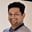Related Tags

java

# How to retrieve, update and delete an element in a Java arrayTarun Telang

## Introduction

An array is a data structure that holds a fixed number of values in contiguous memory locations. Each value in an array is called an element, and each element has a corresponding index.

The length of an array is fixed when the array is created. To retrieve an item from an array, we need to know the index of the item. Java arrays are zero-based, meaning that the first element in the array is at the index 0 and the last element is at index length - 1.

A Java array with a zero-based index

### Retrieve an item from an array

To retrieve an item from an array, we can use the square brackets notation:

myArray[index]
square bracket notation to retrieve an item in an array

For example, if we have an array named myArray, we can retrieve the first element by using myArray and the second element by using myArray.

If we try to access an item in an array with an index that does not exist, we'll get an ArrayIndexOutOfBoundsException.

### Update an item from an array

To update an element into an array, we can use the same square bracket notation. For example, to update the first element of myArray to the value of the variable newValue, we can use:

myArray = newValue;
updating the first element in an array

### Delete an element from an array

We can delete an element from an array by setting the value of the item to null. For example, if we have an array named myArray, we can delete the first element by using:

myArray = null;
Deleting an element from an array

To clear the content of an array, we can use the following code:

myArray = null;
Removing all elements from the array

### Code example

The code example below demonstrates various operations on an array:

import java.util.Arrays;
class HelloWorld {
public static void main( String args[] ) {
// Retrieve an item from an array
int myArray[] = new int[]{1,2,3};
System.out.println(myArray); // Outputs 1

// Update an item in an array
myArray = new int[]{4, 5, 6};
myArray = 7;
System.out.println(Arrays.toString(myArray)); // Outputs {7,5,6}

// clearing an array
myArray = null;
System.out.println(Arrays.toString(myArray)); // Outputs null
}
}
Various operations on an array

### Code explanation

In the code above, we first create an array of integers named myArray. We then retrieve the first element from the array and print it to the console. Next, we update the entire array with new values and then update the first element to a new value, 7. Finally, we clear the entire array by setting it to null.

RELATED TAGS

java

CONTRIBUTORTarun Telang
RELATED COURSES

View all Courses

Keep Exploring

Learn in-demand tech skills in half the time